CHIRAG GOYAL — Published On May 28, 2021 and Last Modified On February 27th, 2023

## Introduction

A decision tree classifier is a supervised Machine Learning Method and a type of classifier that is one of the simplest algorithms that can be used for both regression and classification problems. Therefore, every aspiring Data Scientist and Machine Learning Engineer must know these questions and answers on Decision Trees.

In this article, we will discuss the most important Decision Tree interview questions,which are helpful to get you a clear understanding of the techniques, and also for Data Science Interviews, which cover all topics from its fundamentals to complex level concepts.

Learning Objectives

• This tutorial will explain one of the most common classifiers in Data Science and Machine Learning – Decision Trees.
• You will understand a decision tree’s purpose, uses, strengths, limitations, and key components, including root nodes, branches, leaves, and decision rules.
• You will also learn the steps involved in constructing a decision tree, including identifying the target variable, selecting the best feature to split on, and repeating the process recursively.

This article was published as a part of the Data Science Blogathon

## Decision Trees Interview Questions & Answers

#### Q1. What is the Decision Tree Algorithm?

A Decision Tree is a supervised machine-learning algorithm that can be used for both Regression and Classification problem statements. It divides the complete dataset into smaller subsets while, at the same time, an associated Decision Tree is incrementally developed.

A machine learning model like a decision tree can be easily trained on a dataset by finding the best splits to make at each node. The Decision Trees’ final output is a Tree with Decision nodes and leaf nodes. A Decision Tree can operate on both categorical and numerical data.

Unlike Deep Learning, Decision Trees are easy to interpret and understand, making them a popular choice for decision-making applications in various industries. You can create a decision tree model with a programming language like Python using the scikit-learn library or with R. code.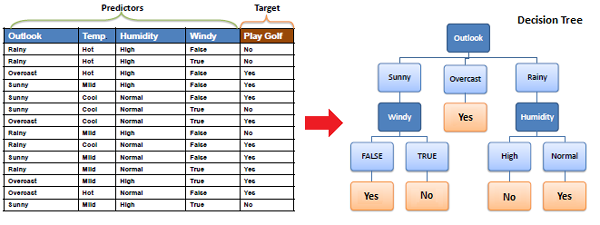#### Q2. List down some popular algorithms used for deriving Decision Trees and their attribute selection measures.

Some of the popular algorithms used for constructing decision trees are:

1. ID3 (Iterative Dichotomiser): Uses Information Gain as an attribute selection measure.
2. C4.5 (Successor of ID3):  UsesGain Ratio as an attribute selection measure.
3. CART (Classification algorithm and Regression Trees) – Uses Gini Index as an attribute selection measure.

#### Q3. Explain the CART Algorithm for Decision Trees.

The CART stands for Classification and Regression Trees, is a greedy algorithm that greedily searches for an optimum split at the top level, then repeats the same process at each of the subsequent levels.

Moreover, it verifies whether the split will lead to the lowest impurity, and the solution provided by the greedy algorithm is not guaranteed to be optimal. It often produces a reasonably good solution since finding the optimal Tree is an NP-Complete problem requiring exponential time complexity.

As a result, it makes the problem intractable even for small training sets. This is why we must choose a “reasonably good” solution instead of an optimal one.

#### Q4. List down the attribute selection measures used by the ID3 algorithm to construct a Decision Tree.

The most widely used algorithm for building a Decision Tree is called ID3. ID3 uses Entropy and Information Gain as attribute selection measures to construct a Decision Tree.

1. Entropy:  A Decision Tree is built top-down from a root node and involves the partitioning of data into homogeneous subsets. To check the homogeneity of a sample, ID3 uses entropy. Therefore, entropy is zero when the sample is completely homogeneous, and entropy of one when the sample is equally divided between different classes.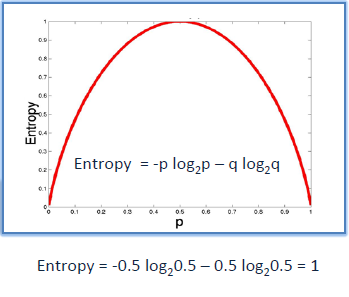2. Information Gain:  Information Gain is based on the decrease in entropy after splitting a dataset based on an attribute. The meaning of constructing a Decision Tree is all about finding the attributes having the highest information gain.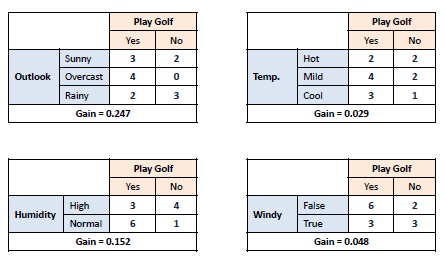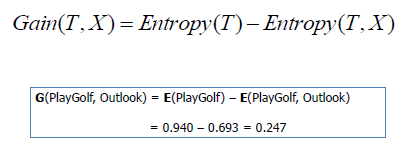#### Q5. Briefly explain the properties of Gini Impurity.

Let X (discrete random variable) takes values y₊ and y₋ (two classes). Now, let’s consider the different cases:

Case- 1:  When 100% of observations belong to y₊ . Then, the Gini impurity of the system would be: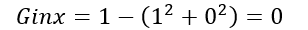Case- 2:  When 50% of observations belong to y₊ . Then, the Gini impurity of the system would be: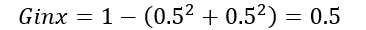Case- 3:  When 0% of observations belong to y₊ . Then, the Gini impurity of the system would be: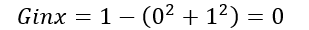After observing all these cases, the graph of Gini impurity w.r.t to y₊ would come out to be: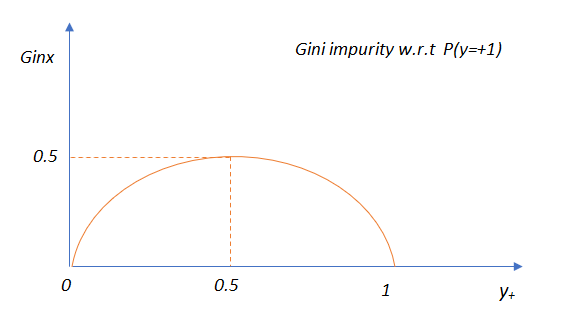#### Q6. Explain the difference between the CART and ID3 Algorithms.

The CART algorithm produces only binary Trees: non-leaf nodes always have two children (i.e., questions only have yes/no answers).

On the contrary, other Tree algorithms, such as ID3, can produce Decision Trees with nodes having more than two children.

#### Q7. Which should be preferred among Gini impurity and Entropy?

In reality, most of the time, it does not make a big difference: they lead to almost similar Trees. Gini impurity is a good default while implementing in sklearn since it is slightly faster to compute. However, when they work differently, then Gini impurity tends to isolate the most frequent class in its own branch of the Tree, while entropy tends to produce slightly more balanced Trees.

#### Q8. List down the different types of nodes in Decision Trees.

The Decision Tree consists of the following different types of nodes:

1. Root node: It is the top-most node of the Tree from where the Tree starts.
2. Decision nodes: One or more decision nodes that result in the splitting of data into multiple data segments. Our main goal is to have the children nodes with maximum homogeneity or purity.
3. Leaf nodes: These nodes represent the data section having the highest homogeneity.

#### Q9. What do you understand about Information Gain? Also, explain the mathematical formulation associated with it.

Information gain is the difference between the entropy of a data segment before and after the split, i.e., reduction in impurity due to the selection of an attribute.

Some points to keep in mind about information gain:

• The high difference represents high information gain.
• The higher the difference implies the lower entropy of all data segments resulting from the split.
• Thus, the higher the difference, the higher the information gain, and the better the feature used for the split.

Mathematically, the information gain can be computed by the equation as follows:

Information Gain = E(S1) – E(S2)

E(S1) denotes the entropy of data belonging to the node before the split.

E(S2) denotes the weighted summation of the entropy of children nodes by considering the weights as the proportion of data instances falling in specific children nodes.

#### Q10. Do we require Feature Scaling for Decision Trees? Explain.

Decision Trees are mainly intuitive, easy to interpret, and require fewer data preparation. In fact, they don’t require feature scaling or centering(standardization) at all. Such models are often called white-box models. Decision Trees provide simple classification rules based on if and else statements that can even be applied manually if necessary.

For Example, Flower classification for the Iris dataset.

#### Q11. What are the disadvantages of Information Gain?

Information gain is defined as the reduction in entropy due to the selection of a particular attribute. Information gain biases the Decision Tree against considering attributes with a large number of distinct values, which might lead to overfitting.

The information Gain Ratio is used to solve this problem.

#### Q12. List down the problem domains in which Decision Trees are most suitable.

Decision Trees are suitable for the following cases:

1. Decision Trees are most suitable for tabular data.
2. The outputs are discrete.
3. Explanations for Decisions are required.
4. The training data may contain errors and noisy data(outliers).
5. The training data may contain missing feature values.

#### Q13. Explain the time and space complexity of training and testing in the case of a Decision Tree.

Time and Space complexity for Training:

In the training stage for features (dimensions) in the dataset, we sort the data, which takes O(n log n) time, following which we traverse the data points to find the right threshold, which takes O(n) time. Subsequently, for d dimensions, the total time complexity would be: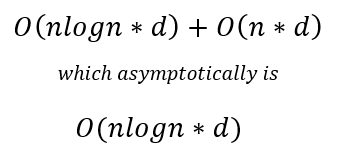Usually, while training a decision tree, we identify the nodes, which are typically stored in the form of if-else statements, due to which training space complexity is O(nodes).

Time and Space Complexity for Testing:

Moreover, the testing time complexity is O(depth) as we have to traverse from the root to a leaf node of the decision tree, i.e., testing space complexity is O(nodes).

#### Q14. If it takes one hour to train a Decision Tree on a training set containing 1 million instances, roughly how much time will it take to train another Decision Tree on a training set containing 10 million instances?

As we know that the computational complexity of training a Decision Tree is given by O(n × m log(m)). So, when we multiply the size of the training set by 10, then the training time will be multiplied by some factor, say K.

Now, we have to determine the value of K. To finds K, divide the complexity of both:

K = (n × 10m × log(10m)) / (n × m × log(m)) = 10 × log(10m) / log(m)

For 10 million instances, i.e., m = 106, then we get the value of K ≈ 11.7.

Therefore, we can expect the training time to be roughly 11.7 hours.

#### Q15. How does a Decision Tree handle missing attribute values?

Decision Trees handle missing values in the following ways:

• Fill the missing attribute value with the most common value of that attribute.
• Fill in the missing value by assigning a probability to each of the possible values of the attribute based on other samples.

#### Q16. How does a Decision Tree handle continuous(numerical) features?

Decision Trees handle continuous features by converting these continuous features to a threshold-based boolean feature. To decide the threshold value, we use the concept of Information Gain, choosing the threshold that maximizes the information gain.

#### Q17. What is the Inductive Bias of Decision Trees?

The ID3 algorithm preferred Shorter Trees over longer Trees. In Decision Trees, attributes with high information gain are placed close to the root and are preferred over those without. In the case of decision trees, the depth of the trees is the inductive bias. If the depth of the tree is too low, then there is too much generalization in the model.

#### Q18. Explain Feature Selection using the Information Gain/Entropy Technique.

The goal of the feature selection while building a Decision Tree is to select features or attributes (Decision nodes) which lead to a split in children nodes whose combined entropy adds up to lower entropy than the entropy value of the data segment before the split. This implies higher information gain.

#### Q19. Compare the different attribute selection measures.

The three measures, in general, returns good results, but:

1. Information Gain: It is biased towards multivalued attributes
2. Gain ratio: It prefers unbalanced splits in which one data segment is much smaller than the other segment.
3. Gini Index: It is biased to multivalued attributes, has difficulty when the number of classes is large, and tends to favor tests that result in equal-sized partitions and purity in both partitions.

#### Q20. Is the Gini Impurity of a node lower or greater than that of its parent? Comment whether it is generally lower/greater or always lower/greater.

A node’s Gini impurity is generally lower than that of its parent as the CART training algorithm cost function splits each of the nodes in a way that minimizes the weighted sum of its children’s Gini impurities. However, sometimes it is also possible for a node to have a higher Gini impurity than its parent. Still, in such cases, the increase is more than compensated by a decrease in the other child’s impurity.

For a better understanding, let’s consider the following Example:

Consider a node containing four samples of class A and one sample of class B.

Then, its Gini impurity is calculated as 1 – (1/5)2 – (4/5)2= 0.32

Now suppose the dataset is one-dimensional, and the instances are arranged in the manner: A, B, A, A, A. We can verify that the algorithm will split this node after the second instance, producing one child node with instances A and B, and the other child node with instances A, A, and A.

Then, the first child node’s Gini impurity is 1 – (1/2)2 – (1/2)2 = 0.5, which is higher than its parent’s. This is compensated for by the other node being pure, so its overall weighted Gini impurity is 2/5 × 0.5 + 3/5 × 0 = 0.2, which is lower than the parent’s Gini impurity.

#### Q21. Why do we require Pruning in Decision Trees? Explain.

After we create a Decision Tree, we observe that most of the time, the leaf nodes have very high homogeneity, i.e., properly classified data. However, this also leads to overfitting. Moreover, if enough partitioning is not carried out, it would lead to underfitting.

Hence, the major challenge is finding the optimal trees that result in the appropriate classification having acceptable accuracy. So to cater to those problems, we first make the decision tree and then use the error rates to prune the trees appropriately. Boosting can also be used to increase the accuracy of the model by combining the predictions of multiple weak learners into a stronger learner.

#### Q22. Are Decision Trees affected by the outliers? Explain.

Decision Trees are not sensitive to noisy data or outliers since extreme values or outliers never cause much reduction in the Residual Sum of Squares(RSS) because they are never involved in the split. Decision Trees are generally robust to outliers. Due to their tendency to overfit, they are prone to sampling errors. If sampled training data is somewhat different than evaluation or scoring data, then Decision Trees tend not to produce great results.

#### Q23. What do you understand by Pruning in a Decision Tree?

The process of removing sub-nodes of a Decision node is called pruning, which is the opposite process of splitting. The two most widely used techniques for pruning are Post and Pre-Pruning.

Post Pruning:

• This type of pruning is used after the construction of the Decision Tree.
• This technique is used when the Decision Tree has a tremendous depth and will show the overfitting of the model.
• It is also known as backward pruning.
• This technique is used when we have an infinitely grown Decision Tree.

Pre Pruning:

• This technique is used before the construction of the Decision Tree.
• Pre-Pruning can be done using Hyperparameter tuning.
• Overcome the overfitting issue.

Note: Below are the advantages and disadvantages of the decision tree algorithm, which is an important interview question on machine learning.

#### Q24. List down the advantages of the Decision Trees.

1. Clear Visualization:  This algorithm is simple to understand, interpret and visualize as the idea is mostly used in our daily lives. The output of a Decision Tree can be easily interpreted by humans.

2. Simple and easy to understand: Decision Tree works in the same manner as simple if-else statements, which are very easy to understand.

3. This can be used for both classification and regression problems.

4. Decision Trees can handle both continuous and categorical variables.

5. No feature scaling required: There is no requirement for feature scaling techniques such as standardization and normalization in the case of a Decision Tree, as it uses a rule-based approach instead of calculating distances.

6. Handles nonlinear parameters efficiently: Unlike curve-based algorithms, the performance of decision trees can’t be affected by non-linear parameters. So, if there is high non-linearity present between the independent variables, Decision Trees may outperform as compared to other curve-based algorithms.

7. Decision Tree can automatically handle missing values.

8. Decision Tree handles the outliers automatically; hence they are usually robust to outliers.

9. Less Training Period: The training period of decision trees is less than that of ensemble techniques like Random Forest because it generates only one Tree, unlike the forest of trees in the Random Forest.

#### Q25. List out the disadvantages of the Decision Trees.

1. Overfitting: This is the major problem associated with Decision Trees. It generally leads to overfitting the data, ultimately leading to wrong predictions for testing data points. It keeps generating new nodes to fit the data, including even noisy data, making the Tree too complex to interpret. In this way, it loses its generalization capabilities. Therefore, it performs well on the training dataset but starts making a lot of mistakes on the test set.

2. High variance: As mentioned, a Decision Tree generally leads to the overfitting of data. Due to the overfitting, there is more likely a chance of high variance in the output, leading to many errors in the final predictions and high inaccuracy in the results. So, achieving zero bias (overfitting) leads to high variance due to the bias-variance tradeoff. You can use an ensemble learning method like Bagging to reduce the prediction variance and make the model more robust to noisy or outlier data points.

3. Unstable: Adding new data points can lead to the regeneration of the overall Tree. Therefore, all nodes need to be recalculated and reconstructed.

4. Not suitable for large datasets: If the data size is large, then one single Tree may grow complex and lead to overfitting. So, in this case, we should use Random Forest instead, an ensemble technique of a single Decision Tree.

#### Q26. What is the role of decision trees in artificial intelligence and machine learning?

Decision trees are simple to interpret and visualize. Also, they can handle both categorical and numerical data. Decision trees can handle non-linear relationships between variables and are robust to outliers. These factors make it a popular choice for various applications in artificial intelligence and machine learning, like natural language processing (NLP), computer vision, and predictive analytics.

#### Q27. How does the decision tree compare with linear regression and logistic regression?

Decision trees are useful in situations where the relationship between the features and the target variable is non-linear and complex. Still, they can easily overfit the data and produce overly complex models. In some cases, decision trees may provide higher accuracy and be more applicable to real-world problems, while in other cases, linear or logistic regression may perform better. The choice of algorithm will depend on the nature of the problem and the data being analyzed.

#### Q28. What are the trade-offs of using decision trees vs. neural networks for certain machine learning applications?

Decision trees are known for their simplicity, interpretability, and ease of use but may not be as accurate as neural networks for complex problems. Neural networks are known for their high accuracy; however, they are computationally intensive, making them more difficult to train and implement than decision trees.

#### Q29. What are some of the alternative algorithms to decision trees?

Some of the alternative algorithms to decision trees are Random Forest, Gradient Boosting, k-Nearest Neighbors (KNN, it labeled data), Logistic Regression, Support Vector Machines (SVMs), Naive Bayes, and Neural Networks.

#### Q30. What common mistakes do beginners make when working with decision trees, and how can you avoid them?

The mistakes beginners would usually make when working with decision trees include overfitting the model to the training data, not properly handling missing values, and not considering the possibility of class imbalance. One can avoid these by using techniques such as pruning the tree, imputing missing values, and performing stratified sampling to balance the class distribution.

## Conclusion

Decision Trees are a supervised learning machine-learning technique that can be used for both regression and classification problems. You create a tree-based model that splits the data into different branches based on a few conditions. The final prediction is made by traversing the tree and making decisions based on the values of the input variables. Decision Trees can be trained using metrics such as Gini Impurity or Entropy, and their performance can be evaluated using metrics such as accuracy, precision, recall, F1 score, and ROC-AUC, confusion matrix(It shows the number of true positive, true negative, false positive, and false negative predictions made by the model).

You can find more machine learning interview questions covering topics such as unsupervised learning, reinforcement learning, k-means, etc., on the AV blog.

Key Takeaways

• The Decision Tree algorithm works by splitting the data into smaller subsets based on the feature values until the data can be split no further into homogeneous groups.
• The final result is a tree-like structure, where each internal node represents a feature, and each leaf node represents the predicted output.
• Decision Trees are simple and allow you to understand the reasoning behind the predictions made by the model. Decision Trees can be prone to overfitting, particularly when dealing with noisy or complex data.
The media shown in this article are not owned by Analytics Vidhya and is used at the Author’s discretion.•